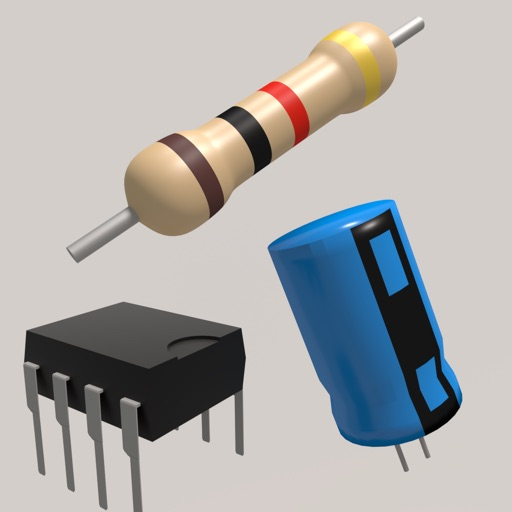## Electronics Toolkit is a helpful app with dozens of tools, calculators and references made for electronic engineers, students and hobbyists# Electronics Toolkit!

by Davey Hollenberg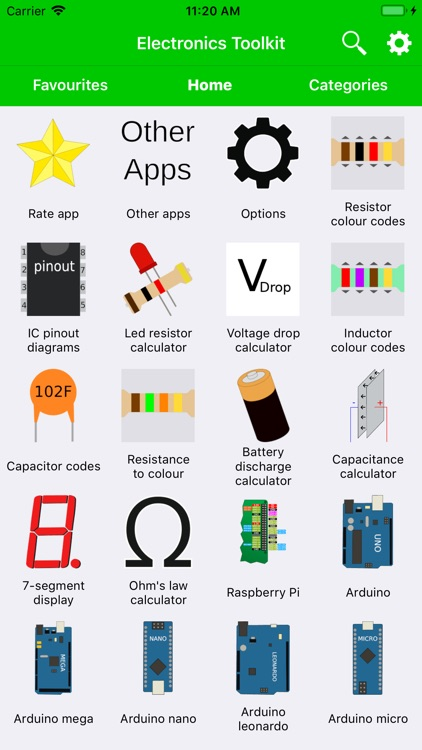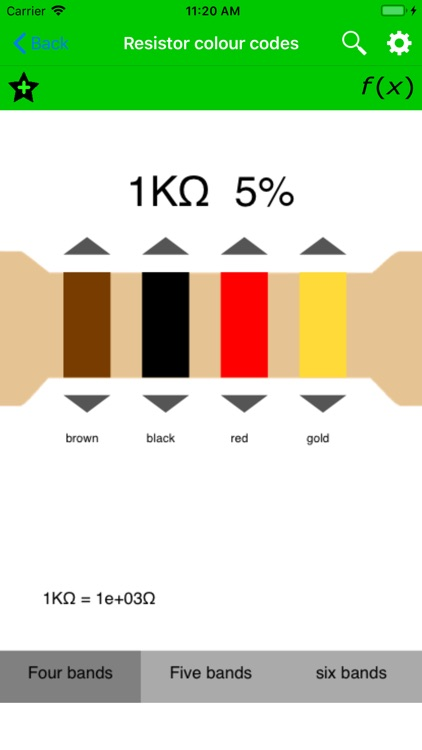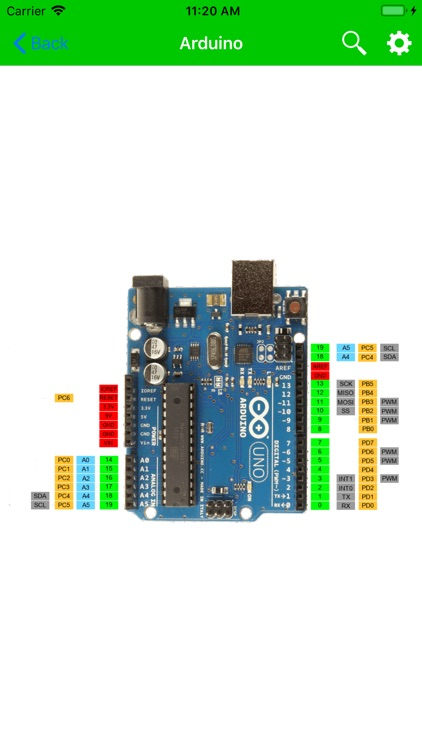Electronics Toolkit is a helpful app with dozens of tools, calculators and references made for electronic engineers, students and hobbyists.### App Details

Version
1.1.5
Rating
(47)
Size
29Mb
Genre
Education Productivity
Last updated
July 20, 2019
Release date
March 2, 2018

### App Screenshots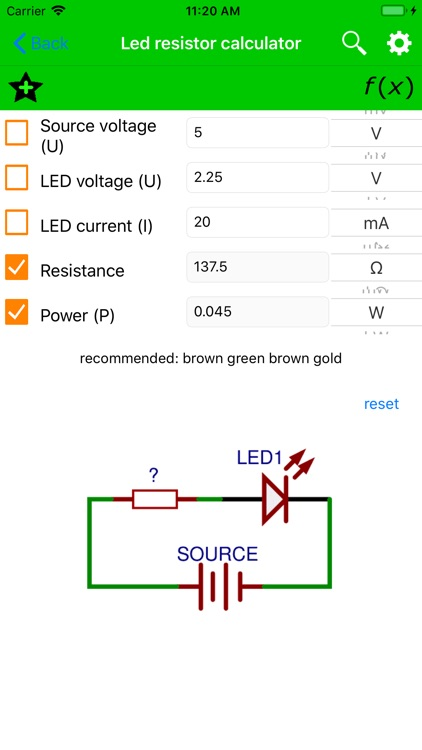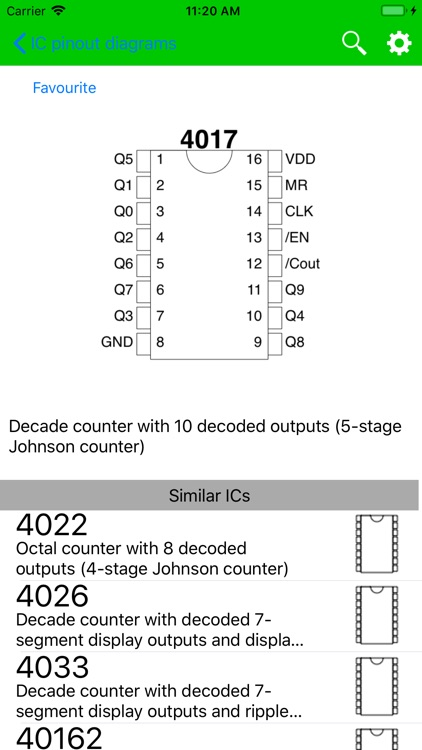### App Store Description

Electronics Toolkit is a helpful app with dozens of tools, calculators and references made for electronic engineers, students and hobbyists.

Features:

Calculators:
• Resistor color codes - calculate the resistance of resistors by selecting the colors of the bands

• SMD resistor codes - calculate the resistance of SMD resistors by entering the number

• LED resistor calculator - calculate the needed resistance to connect en LED to a power source

• Parallel resistors - calculate the resistance of resistors in parallel

• Voltage divider - calculate the output voltage of a voltage divider

• Series resistors - calculate the resistance of resistors in series

• Ohm's law - calculate the voltage, current of resistance by entering the other two

• Capacitance calculator - calculate the capacitance, voltage or charge by entering the other two

• Battery discharge - calculate the time it takes to discharge a battery

• Inductor color codes - calculate the inductance of inductors by selecting the colors of the bands

• Parallel capacitors - calculate the capacitance of capacitors in parallel

• Series capacitors - calculate the capacitance of capacitors in series

• Unit converter - unit converter for length, temperature, area, volume, weight, time, angle, power and base

• Op-amp calculator - calculate the output voltage of non-inverting, inverting, summing and differential opamps

• Wheatstone bridge - calculate the resistance of one resistor in a balanced bridge or calculate the output voltage

• Inductor codes - calculate the inductance of inductors by entering the number

• Capacitor codes - calculate the capacitance of capacitors by entering the number

• DAC and ADC calculator - calculate the output of digital-analog and analog-digital converters

• Wavelength frequency calculator - calculate the frequency or wavelength of a wave

• SI prefixes - convert numbers with SI prefixes

• Capacitor energy - calculate the energy in a capacitor

• Slew rate calculator - calculate the slew rate

• Star delta transformation - calculate the resistors in a star delta transformation

• Zener calculator - calculate the resistance of the resistor and voltage of the zener

• Air core inductor calculator - calculate the inductance and wire length of an air-core inductor

• 555 timer calculator - calculate the frequency, period, duty cycle, high time and low time of a popular 555 timer circuit

• Plate capacitor calculator - calculate the capacity of a plate capacitor

• Resistance to color code calculator - calculate the colors on the resistor by entering the resistance

• LM317 - calculate the output voltage of an LM317

• Low pass filters

• Wire resistance - calculate the resistance of an electrical wire

• RMS voltage

• Decibel calculator

•Reactance

Tables:
• Logic gates - truth table of the 7 logic gates with interactive buttons

• 7-segment display - an interactive display that you can change by clicking on one of the segments or by clicking on a button to show a hexadecimal character

• ASCII - decimal, hexadecimal, binary, octal and char ASCII table

• Resistivity - table with the resistivity of common metals at 293K

• Arduino pinout

• Pinout diagrams of 4000 and 7400 series ICs

Disclaimer:
AppAdvice does not own this application and only provides images and links contained in the iTunes Search API, to help our users find the best apps to download. If you are the developer of this app and would like your information removed, please send a request to [email protected] and your information will be removed.